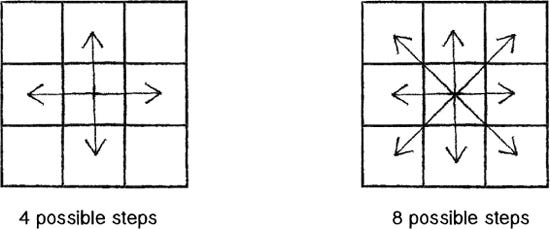I have been offline for around 6 months, and have access to 2G only for the past two weeks or so. There isn’t much one can do on 2G apart from browsing social media, checking emails, and probably blogging (if this post gets uploaded).

I read (and reread) a lot of stuff in 6 months, Harry Potter Fanfics, Fiction, and some programming books. While reading Nature of Code by Daniel Shiffman, I came across a great example for beginners demonstrating how good programming logic can improve the code. He was trying to demonstrate what it means for something to simply move around the screen and also (maybe accidentally) demonstrated efficient coding.

Ever since I read that example, I have been planning to share that with my friends and since I can’t do anything else, you, the reader are also my friend.

I would recommend all beginners to read his book and watch his tutorials on youtube. (I will link to many of his resources at the end).

### What is good/clean code?

I like my code to be elegant and efficient. The logic should be straightforward and make it hard for bugs to hide, the dependencies minimal to ease maintenance, error handling complete according to an articulated strategy, and performance close to optimal so as not to tempt people to make the code messy with unprincipled optimizations. Clean code does one thing well.

Bjarne Stroustrup, inventor of C++Random Walker Output (from Nature of Code Book)

To start, we will first make a Random Walker, an object that moves a step in a random direction after every interval of time.

It will have two pieces of data, its X & Y coordinate, its constructor function, and a function step() to take the next step. (You will also need the function to display it, but I won’t be writing that)

I won’t be writing the entire code, just the part which I need to demonstrate.

``````    class Walker {
int x;
int y;
Walker() {
// Constructor function to initailize data
// width & height have the width & height of the output.
x = width/2;
y = height/2;
}
}``````

I will start with a Walker that can move only in 4 directions (up, down, left, right). To make a random choice from the mentioned 4 choices, I will use a `random(n)` function which returns a random float number between 0 & 4 (0 to 3.9999999….) and use `int(n)` function to remove the decimal part, thus giving us the numbers 0 to 3. Then using simple if-else, I will decide on the direction.

``````    void step(){
int choice = int(random(4));
if(choice == 0){
x++; // Move right
}
else if(choice == 1){
x--; // Move left
}
else if(choice == 2){
y++; // Move up
}
else {
y--; // Move down
}
}``````

This way is probably the most common way to do this, the most you can do is remove the curly brackets from the if-else tree to shorten the code.Now, let’s expand the logic to 9 possible choices, 8 directions (up, up-left, left, down-left, down, down-right, right, up-right) and going nowhere/staying in the same place.

If you don’t have much experience with programming, you probably would expand the code we just wrote to fit in nine choices.

``````    void step(){
int choice = int(random(9));
if(choice == 0){
x++; // Move right
}
else if(choice == 1){
x--; // Move left
}
else if(choice == 2){
y++; // Move up
}
else if(choice == 3){
y--; // Move down
}
else if(choice == 4){
// Move up-right
x++;
y++;
}
else if(choice == 5){
// Move up-left
x--;
y++;
}
else if(choice == 6){
// Move down-left
x--;
y--;
}
else if(choice == 7){
// Move down-right
x++;
y--;
}
// We don’t need to write code for staying
}``````

As you can see, this will make the code a lot longer.

One of the ways to shorten it from 8 blocks of if-else to just 4 blocks is by separating the 9 possibilities into two groups of 3 possibilities. The Walker has 3 choices on X-Axis (left, right or stay) and 3 choices on Y-Axis (up, down or stay). By using two separate random functions, the code will look like:

``````    void step(){
int xchoice = int(random(3));
int ychoice = int(random(3));
if(xchoice == 0){
x++; 	// Move right
}
else if(xchoice == 1){
x--;	// Move left
}
if(ychoice == 0){
y++;	// Move up
}
else if(ychoice == 1){
y--;	// Move down
}
}``````

The last way which I will show is the shortest one requiring 0 if-else blocks, and the method most people would use. If you are good at noticing things and patterns (which you become by practising and writing code), you will see that both X & Y are added 1, subtracted 1 or remain unchanged when step() is called. In other terms, during step() X & Y are added 1 or 0 or -1. We can use this logic to simplify the code to just two lines.

``````    void step(){
x += int(random(3)) - 1; // int(random(3)) — 1; returns -1, 0 or 1
y += int(random(3)) — 1;
}``````

As you can see in this example, there are ways to simplify the code if one can recognise patterns. I am sure the seasoned programmers will know a lot of other such examples (which they could share in the comments, and I will look into editing them into the post later on). For those who have just started learning how to code, I will recommend reading books (especially Nature of Code) and watching other people code or going through their code, to learn other such logics.